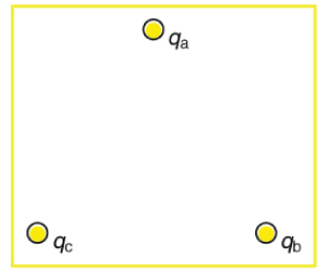# Problem: In the figure, the point charges are located at the corners of an equilateral triangle 22 cm on a side.A.)What is the magnitude of the force in N on Qa given that Qa = 1.3nC?B.)What is the direction of the force on Qa in degrees above the negative x-axis with origin at Qa?

###### FREE Expert Solution

Force:

$\overline{){\mathbf{F}}{\mathbf{=}}\frac{\mathbf{k}{\mathbf{q}}_{\mathbf{1}}{\mathbf{q}}_{\mathbf{2}}}{{\mathbf{r}}^{\mathbf{2}}}}$

Net along the y-direction:

Fb = (9 × 109)(1.3 × 10-9)(11.1 × 10-6)/(22 × 10-2)2 = 2.683 × 10-3 N

F= (9 × 109)(1.3 × 10-9)(5.3 × 10-6)/(22 × 10-2)2 = 1.28 × 10-3 N

Fx = Fb cos (60) + Fc cos (60)

Fx = (2.683 × 10-3) cos (60) + (1.28 × 10-3) cos (60) = 1.982 × 10-3 N

Fy = Fb sin (60) - Fc sin (60)

Fy = (2.683 × 10-3) sin (60) + (1.28 × 10-3) sin (60) = 6.064× 10-2 N

82% (103 ratings)###### Problem Details

In the figure, the point charges are located at the corners of an equilateral triangle 22 cm on a side.

A.)What is the magnitude of the force in N on Qa given that Qa = 1.3nC?

B.)What is the direction of the force on Qa in degrees above the negative x-axis with origin at Qa?